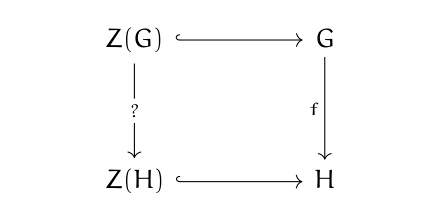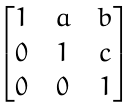# The center may not hold

“… Things fall apart; the centre cannot hold …” — Yeats, The Second Coming

## Center of a group

The center of a group is the set of elements that commute with everything else in the group. For example, matrix multiplication is not commutative in general. You can’t count on AB being equal to BA, though of course sometimes it does, and in fact there are some matrices that commute with all other matrices.

The identity matrix I, for example, commutes with everything. So do multiples of the identity matrix. In fact, those are the only matrices that commute with everything, so the center of the group of invertible n × n matrices consists of multiples of the identity matrix .

## Homorphisms

Now suppose we have a homomorphism f between two groups, G and H. That is, f is a function from G to H such that for any two elements g1 and g2 in G,

f(g1 g2) = f(g1) f(g2).

The function f gives a picture of G inside H that preserves multiplication in the sense above. Does f map the center of G to the center of H? In terms of a diagram, does the following diagram commute?Here the center of a group is denoted with Z(). Why? The convention goes back to the German word zentrum for center. The hooks on the arrows indicate inclusion. If we restrict f to Z(G), is its image contained in Z(H)? Maybe.

## Positive example: Quaternions

A homomorphism may or may not take the center to the center. If a group is Abelian, then the center of the group is the entire group because everything commutes with everything. So then if G and H are Abelian, f takes the center of G (i.e. all of G) to the center of H (i.e. H).

For a less trivial example, let H× be the group of non-zero quaternions under multiplication. Quaternion multiplication is not commutative, so the center Z(H×) is not trivial, and in fact the center is R×, the non-zero real numbers. Let q be a quaternion of unit length and let q* be its conjugate. Define f to be a rotation

f: pqpq*

Then f is a homomorphism and it takes the center, i.e. non-zero real numbers, to itself because if r is real then

qrq* = rqq* = r.

Now for an example where the center does not hold.

## Negative example: Heisenberg matrices

Consider the group of matrices of the formI’ve gotten a little ahead of myself by calling the set of such matrices a group, but in fact it is a group, and it’s known as the Heisenberg group.

The center of the Heisenberg group is the set of matrices of the form above where a = c = 0, matrices with 1s on the diagonal, a possibly non-zero element in the top right corner, and zeros everywhere else.

Let f be the inclusion map from the Heisenberg group to the group of all invertible 3 × 3 matrices. The image of the center is itself, so it contains matrices with non-zero elements in the upper right corner. But as we said at the top of the post, the center of the group of invertible matrices is the set of diagonal matrices (with all diagonal elements equal) and so it doesn’t contain matrices with non-zero elements off the main diagonal.

## Related posts

 To be precise, let K be a field and n a positive integer. The center of the general linear group GLn(K) is the set of elements of the form kI for k in K.

## One thought on “The center may not hold”

1. Now do Auden’s elegy for Yeats:
“…Intellectual disgrace/shines from every human face…”